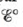# Chlorine dioxide (C1O 2 ), which is produced by the reaction 2 NaC1O 2 ( a q ) + Cl 2 ( g ) → 2ClO 2 ( g ) + 2NaCl ( a q ) has been tested as a disinfectant for municipal water treatment. Using data from Table 17-1, calculate and ∆ G ° at 25°C for the production of ClO 2 .### Chemistry: An Atoms First Approach

2nd Edition
Steven S. Zumdahl + 1 other
Publisher: Cengage Learning
ISBN: 9781305079243

#### Solutions

Chapter
Section### Chemistry: An Atoms First Approach

2nd Edition
Steven S. Zumdahl + 1 other
Publisher: Cengage Learning
ISBN: 9781305079243
Chapter 17, Problem 49E
Textbook Problem
1 views

## Chlorine dioxide (C1O2), which is produced by the reaction 2 NaC1O 2 ( a q )   +  Cl 2 ( g ) → 2ClO 2 ( g ) +  2NaCl ( a q ) has been tested as a disinfectant for municipal water treatment. Using data from Table 17-1, calculateand ∆G° at 25°C for the production of ClO2.

Interpretation Introduction

Interpretation:

The reaction involved in the production of chlorine dioxide (ClO2) is given. The value of E° and ΔG° for the given chemical reaction is to be calculated.

Concept introduction:

The species with higher reduction potential undergoes reduction at the cathode while the species with lower reduction potential undergoes oxidation at the anode. The change in Gibbs free energy is the useful amount work that can be done and negative value of ΔG° shows that the reaction is spontaneous in nature.

To determine: The value of E° and ΔG° for the given reaction.

### Explanation of Solution

The value of E° is calculated as 0.41V_ .

The reaction taking place at cathode is,

Cl2(g)+2e2Cl(aq)E°red=1.36V

The reaction taking place at anode is,

2ClO2(aq)2ClO2(aq)+2eE°ox=0.954V

Add both the oxidation and reduction half-reaction,

2ClO2(aq)+Cl2(g)2ClO2(aq)+2Cl(aq)

The overall cell potential is calculated as,

E°cell=E°ox+E°red=0.954V+1.36V=0.406V0.41V_

The value of E°cell is 0.41V_

### Still sussing out bartleby?

Check out a sample textbook solution.

See a sample solution

#### The Solution to Your Study Problems

Bartleby provides explanations to thousands of textbook problems written by our experts, many with advanced degrees!

Get Started

Find more solutions based on key concepts
Carnitine supplements a. are fat burners that increase cellular energy. b. raise muscle carnitine concentration...

Nutrition: Concepts and Controversies - Standalone book (MindTap Course List)

Which cells utilize fatty acids over glucose to satisfy their energy needs?

Chemistry for Today: General, Organic, and Biochemistry

Determine the density of oxygen at 25C and 741torr.

Introductory Chemistry: An Active Learning Approach

(a) Use the exact result from Example 24.4 to find the electric potential created by the dipole described in th...

Physics for Scientists and Engineers, Technology Update (No access codes included)